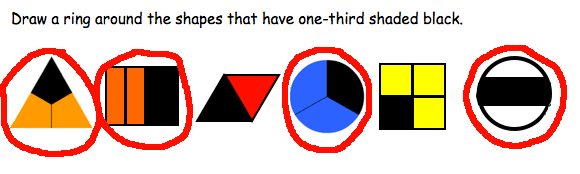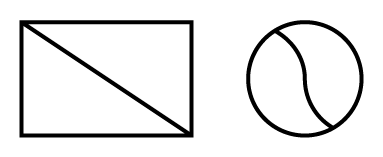# Unequal areas

When asked to choose the shapes that show one-third, a student with the 'number of parts only' misconception has responded by selecting all the shapes that have three parts.Responses to area diagram question.

Typically, students begin their exploration of fractions through using the area model. They are presented with shapes to represent one whole. Students are meant to understand that the area of these shapes can be divided into equal parts to represent fractions.

Difficulties can arise from the early use of the area model for fractions.

• Some students will not yet comprehend the concept of area so will be unsure what is actually being divided into parts.
• Many students will not be ready to compare areas that are different shapes or in different orientations because they have not yet developed the cognitive skill of 'conservation' of area.

The exploration of one-half is often combined with an introduction to line symmetry. While this might assist in understanding symmetry, it might not be as helpful for understanding the concept of half. It is not always the case that the line dividing an area in half will also be a line of symmetry, as in the shapes below.Shapes showing halves but not line symmetry.